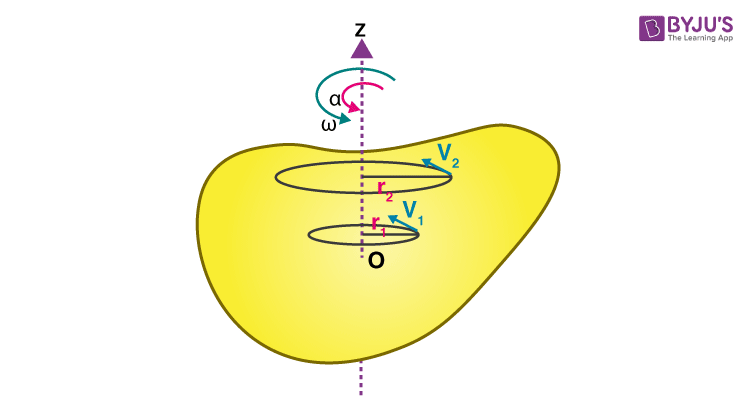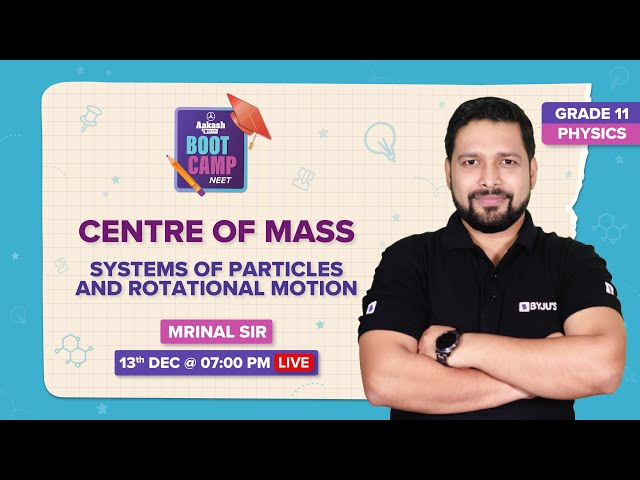# Dynamics Of Rotational Motion About A Fixed Axis

Rigid bodies undergo translational as well as rotational motion. So, in such cases, both the linear and the angular velocity need to be analyzed. In order to simplify these problems, we define the translational and rotational motion of the body separately. In this article, we will be discussing the dynamics of the rotational motion of an object about a fixed axis.

## What is Rotational Motion?

“Rotational motion can be defined as the motion of an object around a circular path, in a fixed orbit.”

The dynamics for rotational motion is completely analogous to linear or translational dynamics. Many of the equations for mechanics of rotating objects are similar to the motion equations for linear motion. In rotational motion, only rigid bodies are considered. A rigid body is an object with a mass that holds a rigid shape.

## Rotational Motion About a Fixed Axis

The figure below shows a rotating body that has a point with zero velocity about which the object undergoes rotational motion. This point can be on the body or at any point away from it. Since the axis of rotation is fixed, we consider only those components of the torques applied to the object that is along this axis as only these components cause rotation in the body. The perpendicular component of the torque will tend to turn the axis of rotation for the object from its position.These results in the emergence of some necessary forces of constraint which finally tends to cancel the effect of these perpendicular components, thus restricting the movement of the axis from its fixed position, rendering its position to be maintained. Since the perpendicular components cause no effect; these components are not considered during the calculation. For any rigid body undergoing a rotational motion about a fixed axis, we need to consider only the forces that lie in planes perpendicular to the axis.

### Why are parallel position vectors not considered?

Forces that are parallel to the axis will give torques perpendicular to the axis and need not be taken into account. Also, only the components of the position vector that are perpendicular to the axis are considered. Components of position vectors along the axis result in torques perpendicular to the axis and thus are not to be taken into account.

## Rotational Motion Examples

### Examples of Rotation about a fixed point

Ceiling fan rotation, rotation of the minute hand and the hour hand in the clock, and the opening and closing of the door are some of the examples of rotation about a fixed point.

### Examples of Rotation about an axis of rotation

Rotation about an axis of rotation includes translational as well as rotational motion. The best example of rotation about an axis of rotation is pushing a ball from an inclined plane. The ball reaches the bottom of the inclined plane through translational motion while the motion of the ball is happening as it is rotating about its axis which is rotational motion.

Another example of rotation about an axis of rotation is the motion of the earth. The earth rotates about its axis every day and it also rotates around the sun once every year. This is a classic example of translational motion as well as rotational motion.

Watch the video and find out how gymnasts increase and decrease their speed while rotating.## Rotational Motion Dynamics

### Moment of Inertia

The moment of inertia is the measure of the object’s resistance to the change in its rotation. The moment inertia is symbolized as I and is measured in kilogram metre² (kg m2.) The moment of inertia is given by the following equations:

I = Mr2, where m is the mass of the particle and r is the distance from the axis of rotation.

The moment of inertia depends on the mass of the particle; the larger the mass the greater the moment of inertia.

Following is the table for a moment of inertia for symmetric bodies:

 Symmetric body Moment of inertia Ring with a symmetric axis $$\begin{array}{l}I = mR^2\end{array}$$ Cylinder or disc with a symmetric axis $$\begin{array}{l}I=\frac{1}{2}mR^{2}\end{array}$$ Uniform sphere $$\begin{array}{l}I=\frac{2}{5}mR^{2}\end{array}$$ Rod with the axis through the end $$\begin{array}{l}I=\frac{1}{3}ml^{2}\end{array}$$ Rod with the axis at the center $$\begin{array}{l}I=\frac{1}{12}ml^{2}\end{array}$$

### Torque

Torque is the twisting effect of the force applied to a rotating object which is at a position r from its axis of rotation. Mathematically, this relationship is represented as follows:

$$\begin{array}{l}\tau =r\times F\end{array}$$

### Angular Momentum

The angular momentum L is a measure of the difficulty of bringing a rotating object to rest. It is given by the following equation:

$$\begin{array}{l}L=\sum r\times p\end{array}$$

## Comparison of Translational Motion and Rotational Motion

The table below lists the quantities associated with linear motion and its analogous in rotational motion:

 Serial Number Parameter Linear Motion Rotational Motion 1 Displacement $$\begin{array}{l}x\end{array}$$ $$\begin{array}{l}\Theta\end{array}$$ 2 Velocity $$\begin{array}{l}v\end{array}$$ $$\begin{array}{l}v=\frac{dx}{dt}\end{array}$$ 3 Acceleration $$\begin{array}{l}a=\frac{dv}{dt}\end{array}$$ $$\begin{array}{l}\alpha =\frac{d\omega }{dt}\end{array}$$ 4 Mass $$\begin{array}{l}M\end{array}$$ $$\begin{array}{l}I\end{array}$$ 5 Force $$\begin{array}{l}F = ma\end{array}$$ $$\begin{array}{l}\tau =I\alpha\end{array}$$ 6 Work $$\begin{array}{l}dW=Fds\end{array}$$ $$\begin{array}{l}W=\tau d\Theta\end{array}$$ 7 Kinetic Energy $$\begin{array}{l}K=\frac{mv^2}{2}\end{array}$$ $$\begin{array}{l}K=\frac{I\omega^2}{2}\end{array}$$ 8 Power $$\begin{array}{l}P=F\nu\end{array}$$ $$\begin{array}{l}P=\tau \omega\end{array}$$ 9 Linear Momentum $$\begin{array}{l}p=MV\end{array}$$ $$\begin{array}{l}L=I\omega\end{array}$$

## Rotational Motion and Work-Energy Principle

According to the work-energy principle, the total work done by the sum of all the forces acting on an object is equal to the change in the kinetic energy of the object.

In rotational motion, the concept of the work-energy principle is based on the torque. It is stated as the object is said to be in a balanced state if its displacements and rotations are equal to zero work when a force is applied.

Consider a rigid body such that Δ𝛳 is the small rotation experienced by the object. Then the linear displacement is given as Δr = rΔ𝛳. This is perpendicular to the r.

Therefore, the work done is

ΔW = F perpendicular to Δr

ΔW = F Δr sin 𝜙

ΔW = Fr Δ𝛳 sin 𝜙

ΔW = 𝜏Δ𝛳

When the number of forces acting is increased, then the work done is given as

ΔW = (𝜏1 + 𝜏2 + ……) Δ𝛳

But we know that Δ𝛳 is the same for all forces.

Therefore, the work done will be zero, that is

𝜏1 + 𝜏2 + …… = 0

Hence, the work-energy principle for rotational motion is proved.

## Relationship Between Torque, Moment of Inertia, and Angular Acceleration

The rotational dynamics can be understood if you have ever pushed a merry-go-round. We observe that the change in the angular velocity of a merry-go-round is possible when a force is applied to it. Another example is the spinning of the bike wheel. As the force is increased, the angular acceleration produced in the wheel would be greater. Therefore, we can say that there is a relationship between the force, mass, angular velocity, and angular acceleration.

Consider a wheel of the bike. Let F be the force acting on the wheel such as angular acceleration produced is 𝛼. Let r be the radius of the wheel. We know that the force is acting perpendicular to the radius. We also know that,

F = ma

Where a is acceleration = r𝛼

Therefore,

F = mr𝛼

We have learned that torque is the turning effect of the force. Therefore,

𝜏 = Fr

rF = mr2𝛼

𝜏 = mr2𝛼

Therefore, we can say that the last equation is the rotational analog of F = ma such that torque is analog of force, angular acceleration is analog of acceleration, and rotational inertia that is mr2 is analog of mass. Rotational inertia is also known as moment of inertia.

The relationship between torque, the moment of inertia, and angular acceleration is

net 𝜏 = I𝛼

𝛼 = net 𝜏/I

Where net 𝜏 is the total torque

See the video below to learn problems based on the center of mass.## Frequently Asked Questions – FAQs

### What is Rotational Motion?

Rotational motion can be defined as the motion of an object around a circular path, in a fixed orbit.

### What are the examples of rotational motion about a fixed axis?

Ceiling fan rotation, rotation of the minute hand and the hour hand in the clock, and the opening and closing of the door are some of the examples of rotation about a fixed point.

### What are the examples of rotational motion about an axis of rotation?

The best example of rotation about an axis of rotation is pushing a ball from an inclined plane. The ball reaches the bottom of the inclined plane through translational motion while the motion of the ball is happening as it is rotating about its axis which is rotational motion.

### What is the moment of inertia?

The moment of inertia is the measure of the object’s resistance to the change in its rotation.

### What is Torque?

Torque is the twisting effect of the force applied to a rotating object which is at a position r from its axis of rotation.

### Are torque and moment of inertia similar?

No, torque and moment of inertia are not similar. Torque is dependent on the magnitude and direction of the force and on the application point. Whereas the moment of inertia is dependent on the mass and the axis of rotation.

### Define tangential acceleration.

Tangential acceleration, at is defined as the linear acceleration of a rotating object such that the linear acceleration is perpendicular to the radial acceleration. The SI unit of tangential acceleration is m/s2.

### What is the difference between angular acceleration and tangential acceleration?

Angular acceleration and tangential acceleration are most of the time considered to be similar, but they are not. Angular acceleration is defined as the change in the angular velocity of an object over time whereas tangential acceleration is defined as the change in the linear velocity of an object over time.

### What is the difference between translational and rotational motion?

• The velocity of an object is constant when the object is moving under translational motion whereas the angular velocity of an object varies when the object is moving under rotational motion.
• In translational motion mass of an object is considered whereas in rotational motion moment of inertia of an object is considered.

Test your knowledge on Rotational motion